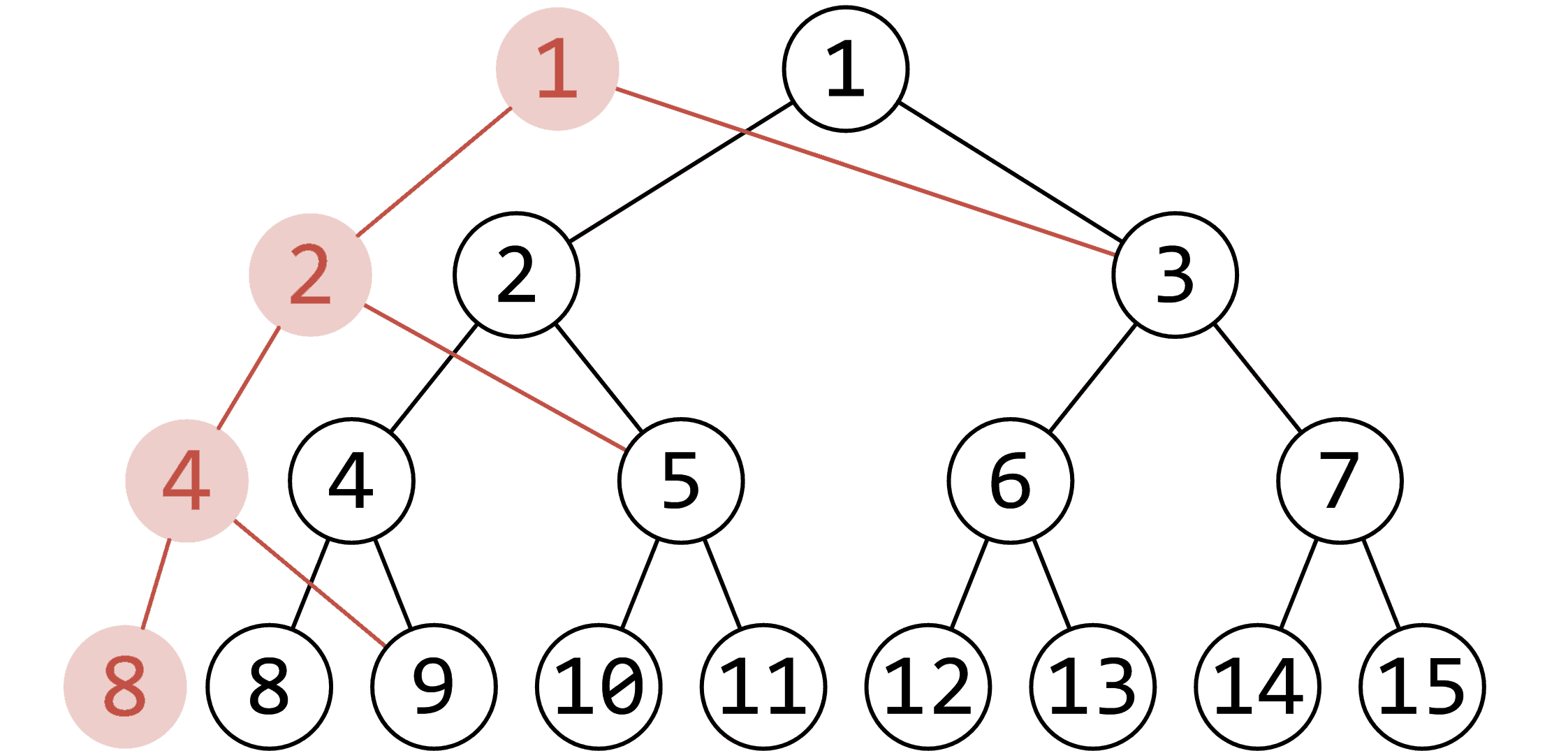# 可持久化线段树

## 主席树¶

（例如下图，修改了 中对应权值为 1 的结点，红色的点即为更改的点）1 2 3 4 5 6 7 8 9 10 11 12 13 14 15 16 17 18 19 20 21 22 23 24 25 26 27 28 29 30 31 32 33 34 35 36 37 38 39 40 41 42 43 44 45 46 47 48 49 50 51 52 53 54 55 56 57 58 59 60 61 #include #include #include using namespace std; const int maxn = 1e5; // 数据范围 int tot, n, m; int sum[(maxn << 5) + 10], rt[maxn + 10], ls[(maxn << 5) + 10], rs[(maxn << 5) + 10]; int a[maxn + 10], ind[maxn + 10], len; inline int getid(const int &val) { // 离散化 return lower_bound(ind + 1, ind + len + 1, val) - ind; } int build(int l, int r) { // 建树 int root = ++tot; if (l == r) return root; int mid = l + r >> 1; ls[root] = build(l, mid); rs[root] = build(mid + 1, r); return root; // 返回该子树的根节点 } int update(int k, int l, int r, int root) { // 插入操作 int dir = ++tot; ls[dir] = ls[root], rs[dir] = rs[root], sum[dir] = sum[root] + 1; if (l == r) return dir; int mid = l + r >> 1; if (k <= mid) ls[dir] = update(k, l, mid, ls[dir]); else rs[dir] = update(k, mid + 1, r, rs[dir]); return dir; } int query(int u, int v, int l, int r, int k) { // 查询操作 int mid = l + r >> 1, x = sum[ls[v]] - sum[ls[u]]; // 通过区间减法得到左儿子的信息 if (l == r) return l; if (k <= x) // 说明在左儿子中 return query(ls[u], ls[v], l, mid, k); else // 说明在右儿子中 return query(rs[u], rs[v], mid + 1, r, k - x); } inline void init() { scanf("%d%d", &n, &m); for (int i = 1; i <= n; ++i) scanf("%d", a + i); memcpy(ind, a, sizeof ind); sort(ind + 1, ind + n + 1); len = unique(ind + 1, ind + n + 1) - ind - 1; rt = build(1, len); for (int i = 1; i <= n; ++i) rt[i] = update(getid(a[i]), 1, len, rt[i - 1]); } int l, r, k; inline void work() { while (m--) { scanf("%d%d%d", &l, &r, &k); printf("%d\n", ind[query(rt[l - 1], rt[r], 1, len, k)]); // 回答询问 } } int main() { init(); work(); return 0; } 

## 参考¶

https://en.wikipedia.org/wiki/Persistent_data_structure

https://www.cnblogs.com/zinthos/p/3899565.html